﻿ Calculation of Electromotive Force in Redox Processes

### Calculation of Electromotive Force in Redox Processes

Algirdas SulciusOPEN ACCESSPEER-REVIEWED

## Calculation of Electromotive Force in Redox Processes

Algirdas SulciusDepartment of Physical and Inorganic Chemistry, Kaunas University of Technology, Lithuania

### Abstract

Chemistry programs at universities include oxidation-reduction (redox) reactions. Based on particular examples the article shows the possibility of application of electromotive force (EMF) calculation to evaluate the possibility for redox reactions to take place and to justify the experimental data. The standard potentials are used for the calculation of EMF of redox processes according to the formula, However, the standard conditions differ from the conditions under which the real experiment is carried out. Therefore, instead of the standard potentials the author of the paper suggests to use stationary metal potentials which have proved them in practice. The formula for calculation of EMF is replaced by the modified formulas: when metals are dissolving in acids and bases;in case of galvanic cells and corrosion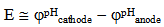. These modified formulas enable more real and precise calculation of EMF of redox processes, what is proved by the provided examples.

• Sulcius, Algirdas. "Calculation of Electromotive Force in Redox Processes." World Journal of Chemical Education 2.2 (2014): 21-25.
• Sulcius, A. (2014). Calculation of Electromotive Force in Redox Processes. World Journal of Chemical Education, 2(2), 21-25.
• Sulcius, Algirdas. "Calculation of Electromotive Force in Redox Processes." World Journal of Chemical Education 2, no. 2 (2014): 21-25.

 Import into BibTeX Import into EndNote Import into RefMan Import into RefWorks

### 1. Introduction

In chemistry, the important role belongs to the redox reactions. They cover a wide range of processes and phenomena that occur in the world around us: respiration and photosynthesis include oxidation and reduction, incineration processes provide the bulk of energy use and transport and in such fields as chemical energy, metallurgy, and electrolysis redox plays a key role. It is impossible to understand the modern inorganic chemistry without examining the redox reactions.

Compiling equations of redox reactions is an integral part of the theoretical study of redox processes. When studying the redox reactions, a number of tasks which require justification for its decision must be fulfilled:

•  selection of an oxidizing agent and a reducing agent;

•  characterization of oxidizing and reducing agents as strong, weak, and so on;

•  evaluation of the possibility for the process to take place;

•  identification of the final forms of the reaction products.

As a rule, these tasks are addressed and resolved by remembering the answers. As for conceptualizing solutions the students do not have the required knowledge, therefore the reference data can be used.

In order to solve the above problems, we use the data presented in the form of three different reference data: table of standard electrode potentials, Latimer‘s diagram and Frost‘s diagram . Each of them has advantages and disadvantages.

The standard electrode potentials enable us to measure oxidizing and reducing agents, compare them, but do not give information required for writing the redox reaction in a variety of environments. For this purpose it is useful to use Latimer’s diagram. Latimer’s diagram represents a sequence of different forms of compounds in varying oxidation degrees and environments of varying acidity. On the Latimer’s diagram, the values of electrode potentials for a particular environment are specified as well and it provides an equation to prove possibility for the redox process to take place in a given environment. However, it is harder to construct the partial redox reaction on the Latimer’s diagram.

It is much more convenient to identify the reaction products on the Frost diagram, which provided the clear information which compound is more stable in the given environment.

IUPAC defines the standard conditions (STP) when the pressure is p = 1.0 • 105 Pa (1 bar), and the temperature is 273.15 K . If the system contains gaseous reagents and/or products and the pressure of each of them is equal to 1.0 • 105 Pa, in aqueous solution the requirement for a standard concentration of the oxidized and the reduced forms, is added and the concentration of each of these forms should be 1 mol/l . For example, in redox reactions of the process of metal dissolution in acids, the acid concentration and the temperature change. The reaction rate is determined by the difficulty of formation of the gas phase and by the solubility of gas in the solution .

It is believed that if Eo = φooxid. agent − φoreduc. agent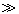0.4 V the redox reaction proceeds to the end . If the value of Eo is in the range of 0.4 - +0.4 V, under STP, the redox reaction will take place with a small reaction rate. In practice such reactions are moving away from the standard conditions and the concentrated solutions of oxidizing and reducing agents must be applied (take the excess of oxidizing agent and a reducing agent, and, if possible, one of the reagents in the form of solid substances or gas). In such cases, the real potential of an oxidizing agent and a reducing agent decreases the potential, which leads to an increase in EMF. In addition, the increasing reaction power sometimes contributes to the increase of the temperature of the reaction mixture. If the Eo = φooxid. agent − φoreduc. agent < 0.4 V, the forward reaction is thermodynamically impossible in any (standard or non-standard) conditions .

This article describes the way to calculate the possibility for the redox processes to take place by using stationary potentials of metals.

### 2. Results and Discussion

2.1. EMF of Metal Dissolution Reaction

Usually, in the redox reactions the more active metal according to the standard potential values (series of metal activity) is determined. However the standard potential is not universal and as a feature can be used only in the reactions of metals with:

– hydrated ions of hydrogen;

– other metal ions (ion concentration 1 mol/l).

The standard potentials are used for the calculation of EMF by applying the formula:

Eo = φooxid. agent − φoreduc. agent. This is clearly described in examples1 and 2.

Example 1

Write the equation of the displacement reaction when copper is placed into silver nitrate solution and specify the valence of the metal in the salt.

Solution

Write the equations of the reactions of displacement:(1)(2)

Find the standard potentials (Table 1):#### Table 1. Potentials of metals in different electrolytesDownload asPowerPoint Slide

Veiw figureView current table in a new window

Calculate the values of EMF for the displacement reactions:Since E01 > E02, we conclude that copper(II) nitrate Cu(NO3)2 is formed.

Example 2

The saturated solution of FeCl3 is used for etching panels. Write the equation of the oxidation-reduction reaction of etching.

Solution

Write the equations of the reactions of displacement: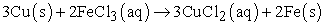(1)(2)

Find the standard potentials (Table 1):Calculate the values of EMF for the displacement reactions: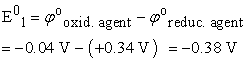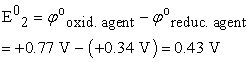Conclusion: the reaction 1 is impossible, and the reaction 2 can take place.

By varying the concentration of the substances involved in the redox process, and adjusting the pH of the solution, one can not only change the voltage response, but also its direction (if the potentials of the two partial reactions are not too different). The standard potentials are useful for studying corrosion processes, but they give only an overall picture, as they do not evaluate the influence of the corrosive environment (pH, oxygen concentration, impurities) on the activity (potential) of metals. For the understanding of corrosion processes the standard potentials cannot be used for two reasons.

1. The surface of metals often quickly is covered by a film of oxides/hydroxides, which reduces the activity of the metal, and to some extent protects the metal. This affects the value of the potential;

2. The concentration (activity) of the ions of the metal in the corrosive solution is much lower than 1 mol/l, which also changes the metal potential value.

Since the real conditions and the standard conditions differ due to many factors (e.g., temperature and pH, concentrations of hydrogen and oxygen in the environment, etc.) the value of the potential is affected . Therefore, at the Department of Physical and Inorganic Chemistry, Kaunas University of Technology the stationary (corrosion) metal potentials (Table 1) are used to calculate the EMF (for example, dissolution of metals in acids). In such case, the formula for the calculation of EMF E0 = φooxid. agent − φoreduc. agent is transformed into the formula E ≅ φpHoxid.agent − φpHreduc.agent.

Application of the modified formula is illustrated by calculation of EMF for the copper dissolution reaction in the concentrated sulphuric acid:The complexity of the reaction of dissolution of copper in the concentrated sulphuric acid is explained by the possibilities of the proceeding of side reactions: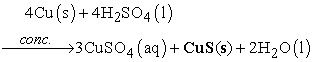(3)(4)

[4, 11](5)(6)(7)(8)



The formation of various compounds when copper is dissolved in the concentrated sulphuric acid can be confirmed by calculating EMF for the reaction:In the real dissolution of copper in the concentrated sulphuric acid, at least two reactions occur: reaction 1 and the secondary reaction 3−8 with the formation of the black precipitate . It is known that the black precipitate contains CuS(s), Cu2S(s) and CuO(s) .

The process of dissolving of metals in acids is influenced by the concentration of the dissolved oxygen in the sulphuric acid, which decreases with the increasing concentration and the temperature of sulphuric acid [12, 14]. By dissolving copper in the concentrated sulfuric acid, reaction 1 takes place, if the concentration of the sulfuric acid does not exceed 92 % . If the concentration of the sulfuric acid is 92−96 % reaction 5, takes place and the light grey crystals of CuSO4(s) are formed . If the concentration of the sulfuric acid is higher than 96 %, a large amount of black CuO(s) precipitates (reaction 6) along with the light grey crystals of CuSO4(s) .

The reaction of basic oxide CuO(s) with sulphuric acid does not take place, since free ions H3O+(aq) are absent in the concentrated (> 96 %) sulphuric acid: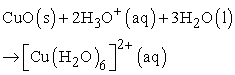(9)



Kinetic of reaction 1, and maybe possibility of formation of different reaction products are also influenced by the shape of copper: copper shapes used cut thin copper wire or copper powder.

The contradicting information about the valence of iron formed by dissolving iron in dilute nitric acid is reported [4, 10]:

1). acid concentration 2050 %(10)



2). acid concentration 5−20 %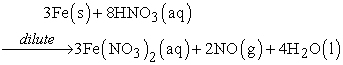(11)



3). acid concentration < 5 %(12)



(or ammonia NH3 (g))

Let us try to calculate EMF of these reactions.

Solutions

1)(10)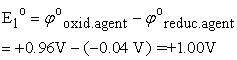Conclusion: under STP the reaction takes place.

2)(11)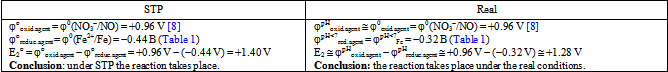3)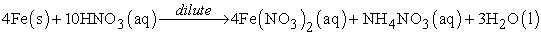(12)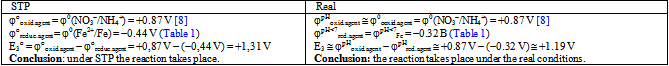The comparison of the vales of EMF shows, that E2o > E3o > E1o (1.40 V > 1.31 V > 1.00 V). Therefore, it is more likely that Fe(II) nitrate, but not Fe(III) nitrate is formed in the dilute nitric acid.

2.2. EMF of Galvanic Cells

Sanger  proposed to use formula EGCo = φocathode − φoanode instead of Eo = φooxid.agent − φoreduc.agent for the calculation of the redox reactions in galvanic cells (GC). The formula is designed for the calculation of EMF for Daniel-Jakobi type GC only. Based on the information mentioned above and given in the paper , the modified formula EGC ≅ φpHcathode − φpHanode which enables to calculate EMF of GC more accurately and more realistically, is used at the Department of Physical and Inorganic Chemistry, Kaunas University of Technology for the corrosion GC i.e. the Volta type GC (see the example).

Example

A piece of zinc is immersed into the solution of hydrochloric acid. For the increase of the solubility the small amount of CuSO4 solution is added. Zinc is coated by copper and the galvanic cell starts to work. Calculate the electromotive force of the galvanic cell. Can copper sulphate be changed by AgNO3? Write the conditional scheme of the galvanic cell formed after the addition of the chosen salt. Write the equations of the reactions which occur on the electrodes and calculate the electromotive force. In which galvanic cell will zinc oxidize faster?

Solution### 3. Conclusions

The table of stationary potentials allows calculating more precisely the electromotive force of reactions of metals dissolution in acids.

In the real situation, when copper dissolves in the concentrated sulphuric acid, at least two reactions occur: reaction with formation of copper sulphate and the secondary reaction with the formation of the black precipitate mainly CuS(s) and CuO(s).

When using the standard potentials of metals, it is impossible to predict which electrode will be anode and which one - the cathode in galvanic cell, for example, Sn/Fe in an alkaline solution, and precisely calculate the electromotive force of galvanic cell. The table of stationary potentials enables more precise calculation of electromotive force of galvanic cell, even for cells like Sn/Fe in an alkaline solution where Sn is the anode. The experimental electromotive force and the one calculated according to the modified formula EGC ≅ φpHcathode − φpHanode differ slightly by electromotive force value for Zn/Cu and Zn/Ag galvanic cells.

### References

  Shriver, D., Atkins P. Inorganic chemistry, W.H. Freeman and Company, 2006, p.851.In article  McNaught A. D., Wilkinson A. Compendium of Chemical Terminology, The Gold book (2nd ed.). Blackwell Science, 1997. Available: http://www.iupac.org/goldbook/S05910.pdf.In article  Lidin, R. A., Savinkina, E. V., Ruk, N. S., Alikperova, L. Ju., Tests of inorganic and general chemistry with solutions (In Russian), Binom, Moscow, 2010, p.230.In article  Drozdov, A.A., Zlomanov, V. P., Mazo, G. M., Inorganic chemistry, Vol. 2 (In Russian), Academy, Moscow, 2004, p. 399.In article  Sulcius A., “Interpretation of voltaic cells in chemistry education”, Journal of Science Education, Vol. 9, No. 2, 114-116. 2008.In article  Stransbury, E. E., Buchanan, R. A., Fundamentals of electrochemical corrosion, ASM International, Ohio, 2000, p. 489.In article  Petrucci R., General chemistry. Principles and modern applications, McMillan Publishing Company, New York, 1989, p. 1042.In article  Dobos, D., Elctrochemical data. A handbook for electrochemists in industry and universities, Kiado, Budapest, 1978, p. 365.In article  Chambers, C.A., Holliday, K. Modern inorganic chemistry, Butterwoth&Co, Great Britain, 1975, p. 405.In article  Tretyakov, Ju. D., Martinenko, L. I., Grigoryev, A. N., Chivadze, A. Yu., Inorganic chemistry. Chemistry of elements, Vol. 2 (In Russian), MGU, Moscow, 2007, p. 670.In article  Lidin, P. A., Molochko, V. A., Andreyeva, L. L., Chemical properties of inorganic compounds (In Russian), Khimiya, Moscow, 2000, p. 286.In article  Grishina, E. P., Udalova, A. M., Rumyantsev, E. M., „Anodic oxidation of copper in concentrated sulfuric acid solutions“, Russian Journal of Electrochemistry, Vol. 38, No. 9, p. 1041-1044, 2002.In article CrossRef  Рипан, Р., Четяну, И., Неорганическая химия. Химия металлов, Т. 1, Мир, Москва, 1972, p. 871.In article  Handbook on Solubility, vol. 3: Triple and Multicomponent Inorganic Systems, Nauka, Leningrad, vol. 1, 1969, p. 36.In article  [Online]. Available: http://www.nationalstemcentre.org.uk/dl/455a39e5f435302500978c4ec141f6bd62f757bd/28823-CCE-39-ReactionBetweenAMetalOxideAndDiluteAcid.pdf.In article  Sanger, M. J., Greenbowe, T. J., An Analysis of College Chemistry Textbooks As Sources of Misconceptions and Errors in Electrochemistry“, Journal of chemical Education, Vol. 76, N. 6, 853-860, 1999.In article CrossRef